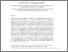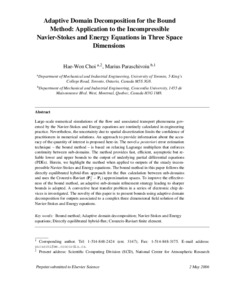Title:

# Adaptive domain decomposition for the bound method: Application to the incompressible Navier–Stokes and Energy equations in three space dimensions

Choi, Hae-Won and Paraschivoiu, Marius (2007) Adaptive domain decomposition for the bound method: Application to the incompressible Navier–Stokes and Energy equations in three space dimensions. Computer Methods in Applied Mechanics and Engineering, 196 (8). pp. 1484-1497. ISSN 0045-7825Preview
Text (application/pdf)
CP2007.pdf - Submitted Version
920kB

Official URL: http://dx.doi.org/doi:10.1016/j.cma.2006.03.015

Divisions: Concordia University > Gina Cody School of Engineering and Computer Science > Mechanical and Industrial Engineering Article Yes Choi, Hae-Won and Paraschivoiu, Marius Computer Methods in Applied Mechanics and Engineering January 2007 10.1016/j.cma.2006.03.015 Bound method; Adaptive domain decomposition; Navier–Stokes and Energy equations; Directly equilibrated hybrid-flux; Crouzeix–Raviart finite element 6754 ANDREA MURRAY 02 Jul 2010 17:20 18 Jan 2018 17:29

## References:

 M. Ainsworth and J.T. Oden, A unified approach to a posteriori error estimation using element residual methods, Numer. Math. 65 (1993), pp. 23–50.

 M. Ainsworth and J.T. Oden, A Posteriori Error Estimation in Finite Element Analysis, Wiley & Sons Inc (2000).

 I. Babuška and W.C. Rheinboldt, A posteriori error estimates for the finite element method, Int. J. Numer. Methods Engrg. 12 (1978), pp. 1597–1615.

 I. Babuška and T. Strouboulis, The Finite Element Method and its Reliability, Oxford University Press (2001).

 R. Becker and R. Rannacher, A feed-back approach to error control in finite element methods: basic analysis and examples, East–West J. Numer. Math. 4 (1996), pp. 237–264.

 R. Becker and R. Rannacher, An optimal control approach to a posteriori error estimation in finite element methods, Acta Numer. 10 (2001), pp. 1–102.

 F.H. Bertrand, M.R. Gadbois and P.A. Tanguy, Tetrahedral elements for fluid flow, Int. J. Numer. Methods Engrg. 33 (1992), pp. 1251–1267.

 G.F. Carey and J.T. Oden, Finite Elements: Fluid Mechanics vol. 4, Prentice-Hall, Englewood Cliffs (1986).

 Z. Cheng and M. Paraschivoiu, Parallel computations of finite element output bounds for conjugate heat transfer, Finite Elem. Anal. Des. 39 (2003), pp. 581–597.

 Z. Cheng and M. Paraschivoiu, A posteriori finite element bounds to linear functional outputs of the three dimensional Navier–Stokes equations, Int. J. Numer. Methods Engrg. 61 (2005), pp. 1835–1859.

 H.-W. Choi, A posteriori finite element bounds with adaptive mesh refinement: application to outputs of the three dimensional convection–diffusion equation, Master’s thesis, Department of Mechanical and Industrial Engineering, University of Toronto, November 2001.

 H.-W. Choi and M. Paraschivoiu, A posteriori finite element output bounds with adaptive mesh refinement: application to a heat transfer problem in a three-dimensional rectangular duct, Comput. Methods Appl. Mech. Engrg. 191 (2002), pp. 4905–4925.

 H.-W. Choi and M. Paraschivoiu, Adaptive computations of a posteriori finite element output bounds: a comparison of the “hybrid-flux” approach and the “flux-free” approach, Comput. Methods Appl. Mech. Engrg. 193 (2004), pp. 4001–4033.

 H.-W. Choi and M. Paraschivoiu, A faster “Hybrid-Flux” calculation approach to output bounds for three dimensional problems, Int. J. Comput. Fluid Dyn. 19 (2005), pp. 115–130.

 H.-W. Choi and M. Paraschivoiu, Advanced hybrid-flux approach for output bounds of electro-osmotic flows: adaptive refinement and direct equilibration strategies, Int. J. Microfluidics Nanofluidics 2 (2006) (2), pp. 154–170.

 R. Glowinski, Finite element methods for incompressible viscous flow. In: P.G. Ciarlet and J.L. Lions, Editors, Handbook of Numerical Analysis vol. IX, North-Holland, Elsevier (2003).

 M.D. Gunzburger, Finite Element Methods for Viscous Incompressible Flows, A Guide to Theory, Practice, and Algorithms, Academic Press, San Diego (1989).

 M.D. Gunzburger, Navier–Stokes equations for incompressible flows: finite-element methods, Handbook of Computational Fluid Mechanics, Academic Press, San Diego (1996) (Chapter 3).

 P. Ladevèze and D. Leguillon, Error estimation procedures in the finite element method and applications, SIAM J. Numer. Anal. 20 (1983), pp. 485–509.

 P. Ladevèze and J.-P. Pelle, Mastering Calculations in Linear and Nonlinear Mechanics, Springer, New York (2005).

 L. Machiels, Y. Maday and A.T. Patera, A “flux-free” nodal Neumann subproblem approach to output bounds for partial differential equations, C.R. Acad. Sci. Ser. I: Math (Numer. Anal.) 330 (2000), pp. 249–254.

 L. Machiels, J. Peraire and A.T. Patera, A posteriori finite element output bounds for the incompressible Navier–Stokes equations: application to a natural convection problem, J. Comput. Phys. 172 (2001), pp. 401–425.

 Y. Maday, A.T. Patera and J. Peraire, A general formulation for a posteriori bounds for output functionals of partial differential equations; application to the eigenvalue problem, C.R. Acad. Sci. Ser. I: Math (Numer. Anal.) 328 (1999), pp. 823–828.

 J.T. Oden and S. Prudhomme, Goal-oriented error estimation and adaptivity for the finite element method, Comput. Math. Appl. 41 (2001), pp. 735–756.

 M. Paraschivoiu, A posteriori finite element output bounds in three space dimensions using the FETI method, Comput. Methods Appl. Mech. Engrg. 190 (2001), pp. 6629–6640.

 M. Paraschivoiu and A.T. Patera, A hierarchical duality approach to bounds for the outputs of partial differential equations, Comput. Methods Appl. Mech. Engrg. 158 (1998), pp. 389–407.

 M. Paraschivoiu, J. Peraire and A.T. Patera, A posteriori finite element bounds for linear-functional outputs of elliptic partial differential equations, Comput. Methods Appl. Mech. Engrg. 150 (1997), pp. 289–312.

 S. Prudhomme and J.T. Oden, On goal-oriented error estimation for elliptic problems: application to the control of pointwise errors, Comput. Methods Appl. Mech. Engrg. 176 (1999), pp. 313–331.

 J.N. Reddy and D.K. Gartling, The Finite Element Method in Heat Transfer and Fluid Dynamics, CRC Press, Boca Raton (1994).

 T. Skalický, LASPack Reference Manual. Institute for Fluid Mechanics, Dresden University of Technology. <http://www.tu-dresden.de/mwism/skalickey/laspack/laspack.html>, 1996. version 1.12.3.

 G. Strang, Introduction to Applied Mathematics, Wellesley-Cambridge Press (1986).

 R. Verfürth, A Review of A Posteriori Error Estimation and Adaptive Mesh-Refinement Techniques, Wiley & Teubner (1996).

 H. Wu, An a posteriori finite element error estimator for adaptive grid computation of viscous incompressible flows, Ph.D. Thesis, Department of Mechanical and Industrial Engineering, University of Toronto, 2000.

 O.C. Zienkiewicz and J.Z. Zhu, A simple error estimator and adaptive procedure for practical engineering analysis, Int. J. Numer. Methods Engrg. 24 (1987), pp. 337–357.
All items in Spectrum are protected by copyright, with all rights reserved. The use of items is governed by Spectrum's terms of access.

Repository Staff Only: item control pageResearch related to the current document (at the CORE website)
Back to top